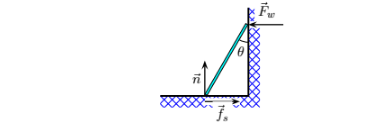# Problem: A uniform bar of mass M and length l is propped against a very slick vertical wall as shown. The angle between the wall and the upper end of the bar is θ. The force of static friction between the upper end of the bar and the wall is negligible, but the bar remains at rest (in equilibrium). If we take the pivot at the point where the bar touches the floor, which expression below is (Στ), where x is along the floor and y is along the wall? 1. ℓ (Fwsinθ + Mg/2 cosθ) = 0 2. ℓ (Mg/2 cosθ − Fwsinθ) = 0 3. ℓ (Mg/2 − Fw) = 0 4. ℓ (−fssinθ − ncosθ) = 0 5. ℓ (Mg/2 + Fw) = 0 6. ℓ (Fwsinθ − Mg/2 cosθ) = 0 7. ℓ (−Mg/2 cosθ − Fwsinθ) = 0 8. ℓ (fssinθ − ncosθ) = 0 9. ℓ (Fwcosθ + Mg/2 sinθ) = 0 10. ℓ (Fwcosθ − Mg/2 sinθ) = 0

🤓 Based on our data, we think this question is relevant for Professor Lai's class at TEXAS.

###### Problem Details

A uniform bar of mass M and length l is propped against a very slick vertical wall as shown. The angle between the wall and the upper end of the bar is θ. The force of static friction between the upper end of the bar and the wall is negligible, but the bar remains at rest (in equilibrium). If we take the pivot at the point where the bar touches the floor, which expression below is (Στ), where x is along the floor and y is along the wall?

1. ℓ (Fwsinθ + Mg/2 cosθ) = 0

2. ℓ (Mg/2 cosθ − Fwsinθ) = 0

3. ℓ (Mg/2 − Fw) = 0

4. ℓ (−fssinθ − ncosθ) = 0

5. ℓ (Mg/2 + Fw) = 0

6. ℓ (Fwsinθ − Mg/2 cosθ) = 0

7. ℓ (−Mg/2 cosθ − Fwsinθ) = 0

8. ℓ (fssinθ − ncosθ) = 0

9. ℓ (Fwcosθ + Mg/2 sinθ) = 0

10. ℓ (Fwcosθ − Mg/2 sinθ) = 0